﻿ MechDesigner Reference & User Interface > Dialog-Boxes > Dialog: Function-Block: Math (with Calculator)

# Dialog: Function-Block: Math (with Calculator)

Navigation:  MechDesigner Reference & User Interface > Dialog-Boxes >

# Dialog: Function-Block: Math (with Calculator)

## Math FB (with Calculator)

See Add Math FB See also :Tutorial 18: Math FB

Use a Math FB to add new math functions.

Use a Math FB to change the Data-Type, (the units of the data). E.g. Change Motion units to Force units, and connect a Math FB to a Spring FB.

#### How to open the Math FB dialog-box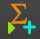To open the Math FB:

 1.Double-click the Math FB in the graphic-area OR 1.

The Math FB dialog-box is now open.

Math FB dialog-box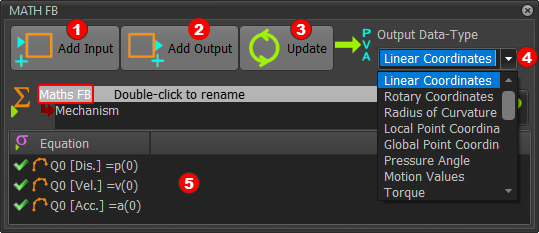#### Math FB Interface

Add Input button1.Click the button to add an input-connectors to the Math FB. You need one input-connector for each variable or parameter in your equation.

Add Output button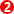1.Click the button to add an output-connector from the Math FB. You need one output-connector for each output.

Update button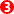1.Click the button when you: •Change the Output Data-Type •Edit an equation

Output Data-Type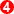The Output Data-Type applies to All of output-connectors from the Math FB. To select a different Output Data-Type 1.Click the drop-down box2.Select the Output Data-Type Notes: Inside the Math FB, all units are SI units. A change to the Data-Type may change the value at the output-connector of the Math FB.

#### Equations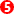There are three(3) Equations for each output-connector See below for more explanations.

Output Data-Type. Output-Connectors, Data-Channels

 The Output Data-Type defines the base units for all of the equations and results of the equations at the output-connectors. Each output-connector has three(3) Data-Channels. You can enter one(1) equation for each Data-Channel. Therefore, you can enter three(3) equations for each output-connector

For example:

If you select Linear Coordinates as the Output Data-Type then ALL equations use Linear Units

If you have two(2) output-connectors, there are 6 equations.

The units for Data-Channels 0 & 3 are Dis, with units of

The units for Data-Channels 1 & 4 are 'Vel', with units of

The units for Data-Channels 2 & 5 are 'Acc', with units of

Data-Channels/Equations 0, 1, 2 are for output-connector 1

Data-Channels/Equations 3, 4, 5 are for output-connector 2

Wire Numbers / input-connectors and Data-Channels

 The image below shows three(3) Wires connected to three(3) Input-Connectors. Each Wire has three Data-Channels.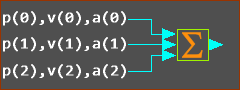3 Wires, 3 Input-Connectors Each wire has 3 Data-Channels Wire-Numbers - refer to the image above. Wire numbers start at 0 •Input-Connector 1, Wire 0 - connected to the TOP input-connector •Input-Connector 2, Wire 1 •Input-Connector 3, Wire 2 - connected to the BOTTOM input-connector Each wire has 3(three) data-channels. Each data-channel is designated with a letter: •p = Data-Channel 1 •v = Data-Channel 2 •a = Data-Channel 3 The format to reference a Wire-Number and Data-Channel in an equation is Data-Channel (Wire-Number) Example - Entries in an Equation: p(0) : p refers to Data-channel 1 ; and (0) refers to input-connector 1 a(2) : a refers to Data-channel 3 ; and (2) refers to input-connector 3 v(1) : v refers to Data-channel 2  ; and (1) refers to input-connector 2

How many input and output-connectors?

As an example, to calculate Power :

P =  Ʈ . ω

Power (N.m./sec) = Torque(N.m.) × Angular Velocity (rad/sec)

Torque data and Angular-Velocity data are inputs to the equation.

Power is the output.

We need two input-connectors and one output-connector.

Edit the Math FB to open the Math FB dialog-box.

2.Select Power for the Output Data-Type.

3.Click UPDATE button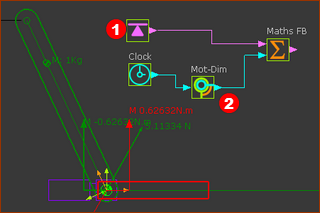#### Prepare the model (see above)

1.Add a Rocker - see Tutorial 1

In CAD-Line dialog-box > User Mass Properties .
Enter a value for the Mass.

Enter X and Y values to move the Center-of-Mass away from the start-Point (origin) of the CAD-Line.

3.Add a Force-Data FB, open the Force-Data FB dialog-box, select the Pin-Joint, and select the Point in the Base-Part to find the Torque that acts-on the Base-Part.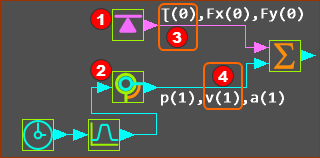See image above - the Data-Channels on the wire of the Force-Data FBand the Motion-Dimension FBThe Math FB uses SI units for all data 'inside'.

#### Enter the Equation

Click the first equation in the Math FB

The Equation-Editor opens - see below.

We need to enter this equation

Q0 [Power] = p(0)*v(1)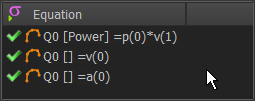p(0) = Wire-Number 0 (top input-connector), Channel 1 (p = Torque)

×

v(1) = Wire-Number 1 (input-connector below top), Channel 2 (v = Angular Velocity)

SYNTAX and VALID Equations

Click the Update after you edit the equation to find out if the syntax is correct

For example, it may not have correct number of parentheses. The reason is given as a message in the Feedback-Area.

The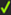at the left of each equation shows if its syntax is correct.

IMPORTANT

Data from Motion FBs

If you connect a wire from a Motion FB to the input of a Math FB, Set the Output Data-Type in the MOTION FB to Linear or Rotary.

### MORE ABOUT UNITS 'inside' the Math FB

 There are 4 possible sources of confusion with respect to 'units' when you use the Math FB. SI Units Inside the Math FB, the units are SI. Example: •If the Motion FB is set to Rotary, and the output from it is 90º, then the value internal to the Math FB is 1.57radians. •If the Motion FB is set to Linear, and the output from it is 100mm, the value internal to the Math FB is 0.1m. If you multiply these together with the Math FB, the internal result is 0.157 - of course. SI Units are converted to Engineering Units The SI units and values that are internal to the Math FB are converted back to Engineering Units of the Output Data-Type. Example continued... •If the Output Data-Type is Linear Coordinates, then 0.157 becomes 157mm at the output-connector when the Engineering Units in the Machine Settings dialog-box are mm. •If the Output Data-Type is Rotary Coordinates, then 0.157 becomes 9º at the output-connector when the Engineering Units in the Machine Settings dialog-box are degrees. The reason? 0.157rads (inside the Math FB) = 9º (outside the Math FB). Linear-Coordinates to a Rocker, Rotary-Coordinates to a Slider Example continued... Rotary units connected to a Linear Slider - that is probably not intended In the Math FB, if you set the Output Data Type to Rotary Coordinates, then 0.157 becomes 0.157rads internally. This becomes 9º at the output, when the Engineering-Units are degrees. If you connect the Math FB to a Motion-Dimension FB to move a Slider, then the 9º becomes 9mm. Linear Units connected to a Rotary Rocker - that is probably not intended In the Math FB, if you set the Output Data Type to Linear Coordinates, then 0.157 is 0.157m internally. This becomes 157mm at the output when the Engineering-Units are mm. If you connect the Math FB to a Motion-Dimension FB to move a Rocker, then the Rocker rotates 157º.

Equation-Editor with Calculator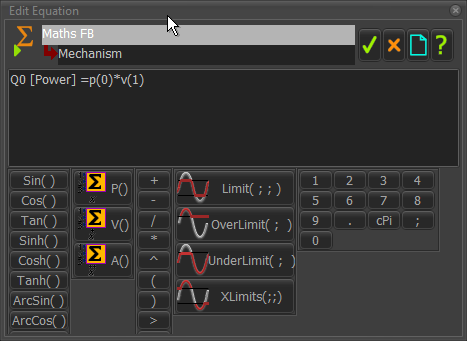Equation-Editor with Calculator

The Equation-Editor provides a few of the common mathematical functions you can add to each equation.

Note 1:

When open and close brackets show within a function button, you must enter a constant value or an variable value at an input-connector.

Example 1: Sin( ),

You can enter a constant - Sin(3.14)

You can also enter a variable - Sin(p(1))  - to calculate the Sine of the continuously varying position values of the second input-connector and wire.

Note 2:

p(0) is data on data-channel 1, at the top, or input-connector #1,

p(1) is data on data-channel 1, at input-connector #2,

p(2) is data on data-channel 1, at input-connector #3, ...

Note 3:

ALL data 'inside' the Math FB are SI units.

#### Calculator Functions

1.Position, Velocity, and Acceleration Values for an linear or angular input

P( ) ; V( ) ; A( )

2.Standard Algebra

Arithmetic: +, –,  ∕ , *

Power: ^

Brackets: ( )

3.Boolean

> : <output> =1 if <value 1> > <value 2>, else 0

< : <output> =1 if <value 1> > <value 2>, else 0

Standard: Sin( ), Cosine( ), Tangent( )

Hyperbolic: Sinh( ), Cosh( ), Tansh( )

Inverse: ArcSin( ), ArcCos( ) , ArcTan2( ; )

5.Limit (input ; maximum value ; minimum value) :

<output> = <input> if <input> is less than maximum value AND more than minimum value.

<output> =  <maximum-value> if <input> is greater than maximum

<output> =  <minimum-value> if <input> is less than minimum

6.OverLimit (input ; limit-value) :

<output> = <input>  if <input> is 'greater than limit-value'

<output> = <limit-value>  if <input> is 'less than limit-value'

7.UnderLimit (input ; limit value) :

<output> = <input>  if <input> is 'less than limit-value'

<output> = <limit-value>  if <input> is 'greater than limit-value'

8.XLimits (input ; maximum value ; minimum value) :

<output> = <input> if <input> is 'greater than maximum value' OR less than minimum value'.

<output> =  <maximum-value> if <input> is 'less than maximum' AND 'more than minimum-value

<output> =  <minimum-value> if <input> is 'greater than minimum-value AND 'less than maximum-value'

10.1...9, 0, Π.

11.Abs( )

12.Mag( ; ) (assumes the two values are 'orthogonal' and uses Pythagoras to find the 'resultant')

13.Sqrt( );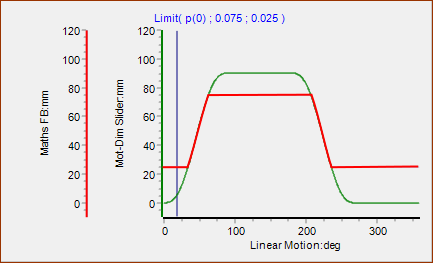Limit Function

Limit (input ; maximum value ; minimum value) :

<output> = <input> if <input> is less than maximum value AND greater than minimum value.

<output> =  <maximum-value> if <input> is greater than maximum

<output> =  <minimum-value> if <input> is greater than minimum

Note that the arguments in the Limit Function are SI units for the units in the model.

E.g.: 75 as millimeters m in the model is 0.075 as meters in the function.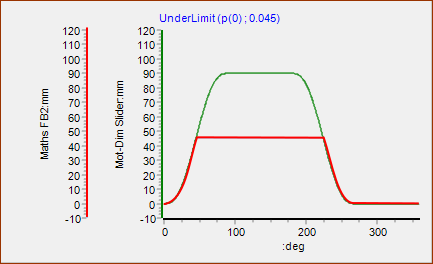Under Limit Function

UnderLimit (input ; limit value) :

<output> = <input>  if <input> is 'less than limit-value'

<output> = <limit-value>  if <input> is 'greater than limit-value'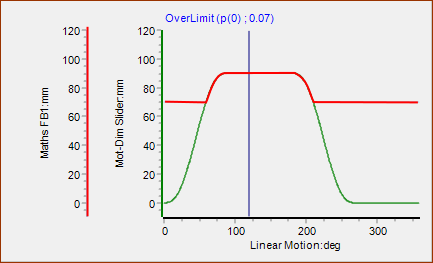Over Limit Function

OverLimit (input ; limit-value) :

<output> = <input>  if <input> is 'greater than limit-value'

<output> = <limit-value>  if <input> is 'less than limit-value'